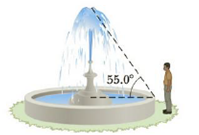Chapter 1, Problem 47P

Chapter
Section
Textbook Problem

A high fountain of water is located at the center of a circular pool as shown in Figure P1.47. Not wishing to get his feet wet, a student walks around the pool and measures its circumference to be 15.0 m. Next, the student stands at the edge of the pool and uses a protractor to gauge the angle of elevation at the bottom of the fountain to be 55.0°. How high is the fountain?Figure P1.47

To determine
The height of the fountain.

Explanation

Given Info: The circumference of the circular pool (C) is 15m and the radius of the circular pool (r) is 2.39m .

Explanation:

The radius of the circular pool (r) .

Formula to calculate the radius of the circular pool is,

C=2πr

• C is circumference of the circular pool,
• r is radius of the circular pool

Substitute 15m for C to find r .

(15m)=2πrr=2.39m

Thus, the radius of the circular pool (r) is 2.39m

The height of the fountain (h)

And the angle θ is 55°

The diagram is given by,

Still sussing out bartleby?

Check out a sample textbook solution.

See a sample solution

The Solution to Your Study Problems

Bartleby provides explanations to thousands of textbook problems written by our experts, many with advanced degrees!

Get Started